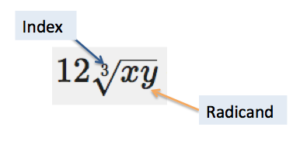### Learning Outcomes

• Recognize when a radical expression can be simplified either before or after addition or subtraction

There are two keys to combining radicals by addition or subtraction: look at the index, and look at the radicand. If these are the same, then addition and subtraction are possible. If not, then you cannot combine the two radicals. In the graphic below, the index of the expression $12\sqrt{xy}$ is $3$ and the radicand is $xy$.Making sense of a string of radicals may be difficult. One helpful tip is to think of radicals as variables, and treat them the same way. When you add and subtract variables, you look for like terms, which is the same thing you will do when you add and subtract radicals.

In this first example, both radicals have the same radicand and index.

### Example

Add. $3\sqrt{11}+7\sqrt{11}$

This next example contains more addends, or terms that are being added together. Notice how you can combine like terms (radicals that have the same root and index), but you cannot combine unlike terms.

### Example

Add. $5\sqrt{2}+\sqrt{3}+4\sqrt{3}+2\sqrt{2}$

Notice that the expression in the previous example is simplified even though it has two terms: $7\sqrt{2}$ and $5\sqrt{3}$. It would be a mistake to try to combine them further! Some people make the mistake that $7\sqrt{2}+5\sqrt{3}=12\sqrt{5}$. This is incorrect because$\sqrt{2}$ and $\sqrt{3}$ are not like radicals so they cannot be added.

### Example

Add. $3\sqrt{x}+12\sqrt{xy}+\sqrt{x}$

Sometimes you may need to add and simplify the radical. If the radicals are different, try simplifying first—you may end up being able to combine the radicals at the end as shown in these next two examples.

### Example

Add and simplify. $2\sqrt{40}+\sqrt{135}$

### Example

Add and simplify. $x\sqrt{x{{y}^{4}}}+y\sqrt{{{x}^{4}}y}$

The following video shows more examples of adding radicals that require simplification.

Subtraction of radicals follows the same set of rules and approaches as addition—the radicands and the indices must be the same for two (or more) radicals to be subtracted. In the three examples that follow, subtraction has been rewritten as addition of the opposite.

### Example

Subtract. $5\sqrt{13}-3\sqrt{13}$

### Example

Subtract. $4\sqrt{5a}-\sqrt{3a}-2\sqrt{5a}$

In the following video, we show more examples of subtracting radical expressions when no simplifying is required.

### Example

Subtract and simplify. $5\sqrt{{{a}^{5}}b}-a\sqrt{16ab}$, where $a\ge 0$ and $b\ge 0$